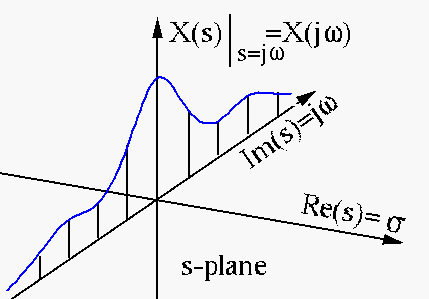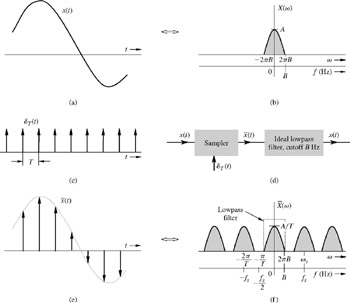# 深入理解傅里叶变换

41 篇文章 2 订阅

## 什么是傅里叶变换

s(x)=A02+n=1Ansin(2πnf0x+ϕn).s(x)=A02+∑n=1∞An⋅sin⁡(2πnf0x+ϕn).f^(ξ)=f(x) e2πixξdx,f^(ξ)=∫−∞∞f(x) e−2πixξdx,## 为什么要分解为正弦波的叠加

d2xdt2+2ω0dxdt+ω20x=F(t).d2xdt2+2ω0dxdt+ω02x=F(t).

x,t,ω0,Fx,t,ω0,F分别代表位移、时间、系统固有频率和外界驱动力。当没有外界驱动力FF时，这个系统有通解

x(t)=Asin(ω0t+ϕ)x(t)=Asin⁡(ω0t+ϕ)

L^x(t)=F(t)L^x(t)=F(t)

L^L^是线性算子，你可以简单地理解为谐振子方程中的左边操作。如果C(t),x0(t)C(t),x0(t)分别是其次方程通解和非齐次方程特解，即他们满足

L^C(t)=0,L^x0(t)=F(t).L^C(t)=0,L^x0(t)=F(t).

L^(x0(t)+C(t))=F(t)L^(x0(t)+C(t))=F(t)

L^xn(t)=sin(wnt),n=0,1...L^nAnxn(t)=nAnsin(wnt).L^xn(t)=sin⁡(wnt),n=0,1...L^∑nAnxn(t)=∑nAnsin⁡(wnt).

w2X^(w)2wω0X^(w)+ω20X^(w)=F^(w)w2X^(w)−2wω0X^(w)+ω02X^(w)=F^(w)

X^(w)=F^(w)(wω0)2X^(w)=F^(w)(w−ω0)2

## 傅里叶变换与量子力学

Φ(p)=12πΨ(x)eipxdx,Ψ(x)=12πΦ(p)eipxdp.Φ(p)=12πℏ∫Ψ(x)e−iℏpxdx,Ψ(x)=12πℏ∫Φ(p)eiℏpxdp.

## 傅里叶变换、拉普拉斯、Z变换、离散傅里叶变换的关系Xsampling(s)=X(s)n=ens/fsXsampling(s)=X(s)∑n=−∞∞ens/fsX(z)=Xsampling(z=es/fs)=X(s=fslnz)n=znX(z)=Xsampling(z=e−s/fs)=X(s=fsln⁡z)∑n=−∞∞zn

X(k)=X(z=ej2πNk)=X(s=j2πNkfs)n=ej2πNnk=X(s=j2πNkfs)=x(t)ej2πNkfstdt=nxnej2πNnkX(k)=X(z=e−j2πNk)=X(s=−j2πNkfs)∑n=−∞∞e−j2πNnk=X(s=−j2πNkfs)=∫−∞∞x(t)e−j2πNkfstdt=∑nxne−j2πNnk

n=ej2πNnk=1x(t)ej2πNkfstdt=nxnej2πNnk∑n=−∞∞e−j2πNnk=1∫−∞∞x(t)e−j2πNkfstdt=∑nxne−j2πNnk

## 傅里叶变换特殊的原因解释

<f,g>=f(t)g(t)dt<f,g>=∫−∞∞f(t)g(t)dt

b=Axb=Ax

[x1,x2,...,xn]=i=1nxiei[x1,x2,...,xn]=∑i=1nxiei

f(t)=f(s)δ(ts)dsf(t)=∫−∞∞f(s)δ(t−s)ds

f(t)=F(w)eiwtdwf(t)=∫−∞∞F(w)e−iwtdw

F(w)就是基函数eiwte−iwt前面的系数。傅里叶变换就是在这两组基函数间的线性变换

aiixi=bi,i=1,...,naiixi=bi,i=1,...,n

λixi=bi,i=1,...,nλixi′=bi′,i=1,...,n

(ddt+a)y(t)=z(t)(ddt+a)y(t)=z(t)

(ddt+a)est=(iw+a)est(ddt+a)est=(iw+a)est

y(t)=Y(w)eiwtdwz(t)=Z(w)eiwtdwy(t)=∫Y(w)eiwtdwz(t)=∫Z(w)eiwtdw

(iw+a)Y(w)=Z(w),wR(iw+a)Y(w)=Z(w),w∈R

2ϕ(x)=ρ(x)∇2ϕ(x)=ρ(x)

G(k,x)=eikxG(k,x)=eik⋅x

(k2x+k2y+k2z)ϕ(k)=ρ(k)−(kx2+ky2+kz2)ϕ(k)=ρ(k)

## 其他微分算子的特征函数举例

L^=k=0nakdkxdtk,akRL^=∑k=0nakdkxdtk,ak∈R

L^=x2d2ydx2+xdydx+(x2α2)L^=x2d2ydx2+xdydx+(x2−α2)

Pn(x)=12nn!dndxn[(x21)n].Pn(x)=12nn!dndxn[(x2−1)n].

L^=ddx[(1x2)ddx]+n(n+1)L^=ddx[(1−x2)ddx]+n(n+1)

Ln(x)=exn!dndxn(exxn)=1n!(ddx1)nxnL^=xd2dx2+(1x)ddx+nLn(x)=exn!dndxn(e−xxn)=1n!(ddx−1)nxnL^=xd2dx2+(1−x)ddx+n

04-27769007-286292
06-092万+
03-24578
01-164万+
11-15
10-101852
06-02点击重新获取扫码支付余额充值